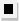Chapter 13.6, Problem 32E### Algebra and Trigonometry (MindTap ...

4th Edition
James Stewart + 2 others
ISBN: 9781305071742

#### Solutions

Chapter
Section### Algebra and Trigonometry (MindTap ...

4th Edition
James Stewart + 2 others
ISBN: 9781305071742
Textbook Problem

# 2 9 - 4 2Terms of a Binomial Expansion Find the indicated terms in the expansion of the given binomial.The first three terms in the expansion of ( x + 1 x ) 40

To determine

To find:

The first three terms in the expansion of (x+1x)40

Explanation

Given:

The binomial expression (x+1x)40

Approach:

Use the formula for expansion of given binomial expression to compute the first three terms.

Calculation:

The binomial expression can be expanded as

(x+1x)40=k=040(40k)(x)40k(1x)k

The first three terms in the expansion given above corresponds to k=0,1,2

So the first three terms are

(400)(x)400(1x)0+(

### Still sussing out bartleby?

Check out a sample textbook solution.

See a sample solution

#### The Solution to Your Study Problems

Bartleby provides explanations to thousands of textbook problems written by our experts, many with advanced degrees!

Get Started